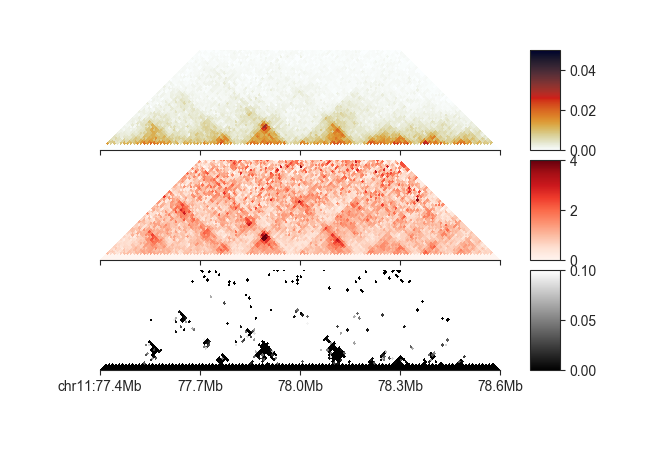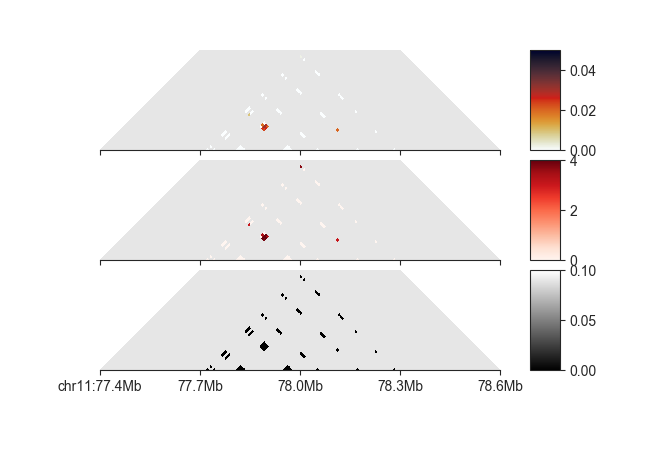# Loop calling¶

To follow this tutorial, download the FAN-C example data, for example through our Keeper library, and set up your Python session like this:

```import fanc
import fanc.peaks
import fanc.plotting as fancplot

import logging
logging.basicConfig(level=logging.INFO, format="%(asctime)s %(levelname)s %(message)s")

```

Also have a look at the command line documentation at Loop calling for the command-line approach to loop calling in FAN-C.

Loop calling in FAN-C is a 3-step process:

1. Annotate pixels with local enrichment, probability, and mappability information
2. Filter pixels based on suitable cutoffs to identify pixels that are part of a loop
3. Merge nearby pixels into a single loop call

## Annotate pixels¶

Start by annotating each pixel with loop information using the `RaoPeakCaller` and `call_peaks()` function:

```loop_caller = fanc.RaoPeakCaller()
loop_info = loop_caller.call_peaks(hic_sample,
file_name="architecture/loops/rao2014.chr11_77400000_78600000.loop_info")
```

This creates a `RaoPeakInfo` object, which contains a bunch of information on each pixel. Here are the most important ones:

• `weight` and `uncorrected` are the normalised and raw contact strength, respectively
• `e_d`, `e_h`, `e_v`, and `e_ll` are the expected values calculated from the donut, horizontal, vertical, and lower left neighborhoods, repectively, of the pixel
• `oe_d`, `oe_h`, `oe_v`, `oe_ll` are the observed/expected (O/E) values calculated from the donut, horizontal, vertical, and lower left neighborhoods, repectively, of the pixel
• `fdr_d`, `fdr_h`, `fdr_v`, `fdr_ll` are the false discovery rates (FDRs) calculated from the donut, horizontal, vertical, and lower left neighborhoods, repectively, of the pixel
• `mappability_d`, `mappability_h`, `mappability_v`, `mappability_ll` are the mappabilities of the donut, horizontal, vertical, and lower left neighborhoods, repectively, of the pixel. This is a value between 0 and 1 stating how many pixels in each neighborhood have valid weights

By default, most intra-chromosomal pixels will receive this information, but you have some influence over the computation with additional parameters. For example, loops tend to occur away from the diagonal, so you may want to exclude pixels near the diagonal using `min_locus_dist`, whihc is expressed in bins. You can also change the values for `p` and `w_init` (see original publication for details) - otherwise sensible defaults are chosen. `min_mappable_fraction` controls which pixels are excluded if their local neighbourhoods are below a certain mappability fraction, which is 0.7 by default, i.e. 70% of each neighbourhood must be valid.

Finally, loops calling is very resource intensive and needs to be heavily parallelised. The number of parallel processes is controlled with `n_processes`. By default, this runs loop calling locally. However, we highly recommend setting `cluster=True` if you are in a cluster environment that supports DRMAA (e.g. SGE, Slurm). You may need to set the `DRMAA_LIBRARY_PATH` environment variable to the location of your `libdrmaa.so` in your shell in order for this to work. `call_peaks()` must then be called from a head node in order to be able to submit jobs to the cluster. Read more about the interface with DRMAA in the gridmap package.

To access the new pixel information, you can use the `peaks()` iterator:

```for edge in loop_info.peaks(lazy=True):
print(edge.oe_d, edge.fdr_d)
```

We can also plot it like a regular matrix:

```# regular Hi-C
p_hic = fancplot.TriangularMatrixPlot(loop_info, vmin=0, vmax=0.05,
max_dist=600000)
# Donut O/E values
p_oe_d = fancplot.TriangularMatrixPlot(loop_info, vmin=0, vmax=4,
weight_field='oe_d', colormap='Reds',
max_dist=600000)
# Donut FDR values
p_fdr_d = fancplot.TriangularMatrixPlot(loop_info, vmin=0, vmax=0.1,
weight_field='fdr_d', colormap='binary_r',
max_dist=600000)

gf = fancplot.GenomicFigure([p_hic, p_oe_d, p_fdr_d], ticks_last=True)
fig, axes = gf.plot('chr11:77.4mb-78.6mb')
```Note how we are controlling which attribute is plotted with `weight_field`.

These kinds of plots can be extremely useful to choose appropriate filtering thresholds for each of these attributes, which we will see in the next section.

## Filter pixels¶

Based on the annotation, we can try to filter out pixels that are not part of loops. This can be done with filters, specifically

Each of these is instantiated and then added to the object using `filter()`. When applying multiple filters it is recommended to first queue them using `queue=True` and to run all of them together with `run_queued_filters()`:

```# filter on O/E
enrichment_filter = fanc.peaks.EnrichmentPeakFilter(
enrichment_ll_cutoff=1.75,
enrichment_d_cutoff=3,
enrichment_h_cutoff=2,
enrichment_v_cutoff=2)
loop_info.filter(enrichment_filter, queue=True)

# filter on FDR
fdr_filter = fanc.peaks.FdrPeakFilter(
fdr_ll_cutoff=0.05,
fdr_d_cutoff=0.05,
fdr_h_cutoff=0.05,
fdr_v_cutoff=0.05)
loop_info.filter(fdr_filter, queue=True)

# filter on mappability
mappability_filter = fanc.peaks.MappabilityPeakFilter(
mappability_ll_cutoff=0.7,
mappability_d_cutoff=0.7,
mappability_h_cutoff=0.7,
mappability_v_cutoff=0.7)
loop_info.filter(mappability_filter, queue=True)

# filter on distance from diagonal
distance_filter = fanc.peaks.DistancePeakFilter(5)
loop_info.filter(distance_filter, queue=True)

# filter on minimum number of observed values
observed_filter = fanc.peaks.ObservedPeakFilter(10)
loop_info.filter(observed_filter, queue=True)

loop_info.run_queued_filters()
```We could also tweak the above cutoffs to further remove noisy pixels. The next step is to merge pixels belonging to the same loop into loop calls.

## Merge pixels¶

To merge the remaining pixels into loops use

```merged_peaks = loop_info.merged_peaks()
```

`merged_peaks` now contains putative loop calls. However, there will still be a number of false-positive loops in this object, which typically consist of a single enriched pixel, likely due to noise. Real loops generally consist of multiple pixels. We can remove the “singlets” using `RaoMergedPeakFilter`:

```singlet_filter = fanc.peaks.RaoMergedPeakFilter(cutoff=-1)
merged_peaks.filter(singlet_filter)
```

A cutoff here of `-1` remove all singlets. In Rao, Huntley et al. (2014) they only remove singlets if their qvalue sum is larger than `0.02`, which you can use instead of `-1` and particularly strong singlets will still be kept in the data.

For our example dataset this leaves only a single loop, which we can export with `to_bedpe()`:

```# regular Hi-C
merged_peaks.to_bedpe("architecture/loops/rao2014.chr11_77400000_78600000.bedpe")
# chr11	77807143	77842857	chr11	77947143	77982857	.	0.0
```